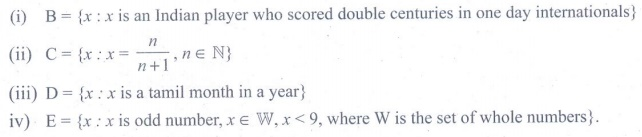Home | | Maths 9th std | Exercise 1.1 : Set

# Exercise 1.1 : Set

Maths : Set Language : Set - Book Back, Exercise, Example Numerical Question with Answers, Solution : Exercise 1.1

Exercise 1.1

1. Which of the following are sets?

(i) The collection of prime numbers upto 100.

(ii) The collection of rich people in India.

(iii) The collection of all rivers in India.

(iv) The collection of good Hockey players.2. List the set of letters of the following words in Roster form.

(i) INDIA (ii) PARALLELOGRAM (iii) MISSISSIPPI (iv) CZECHOSLOVAKIA3. Consider the following sets A = {0, 3, 5, 8}, B = {2, 4, 6, 10} and C = {12, 14,18, 20}.

(a) State whether True or False:

(i) 18 Ōłł C (ii) 6 Ōłē A (iii) 14 Ōłē C (iv) 10 Ōłł B (v) 5 Ōłł B (vi) 0 Ōłł B

(b) Fill in the blanks:

(i) 3 Ōłł ____ (ii) 14 Ōłł _____ (iii) 18 ____ B (iv) 4 _____ B4. Represent the following sets in Roster form.

(i) A = The set of all even natural numbers less than 20.

(ii) B = {y : y = 1/2n , n Ōłł ŌäĢ , n Ōēż 5}

(iii) C = {x : x is perfect cube, 27 < x < 216}

(iv) D = {x : x Ōłł Ōäż , ŌĆō5 < x Ōēż 2}5. Represent the following sets in set builder form.

(i) B = The set of all Cricket players in India who scored double centuries in One Day Internationals.

(ii) C = {1/2, 2/3, 3/4,.... }

(iii) D = The set of all tamil months in a year.

(iv) E = The set of odd Whole numbers less than 9.6. Represent the following sets in descriptive form.

(i) P ={ January, June, July}

(ii) Q = {7,11,13,17,19,23,29}

(iii) R = {x : x Ōłł ŌäĢ , x < 5}

(iv) S = {x : x is a consonant in English alphabets}Tags : Numerical Problems with Answers, Solution | Set Language | Maths , 9th Maths : UNIT 1 : Set Language
Study Material, Lecturing Notes, Assignment, Reference, Wiki description explanation, brief detail
9th Maths : UNIT 1 : Set Language : Exercise 1.1 : Set | Numerical Problems with Answers, Solution | Set Language | Maths